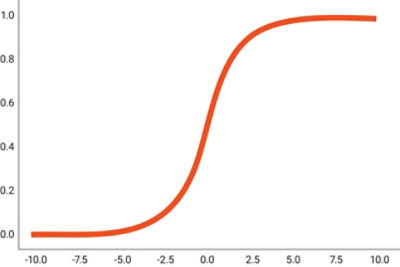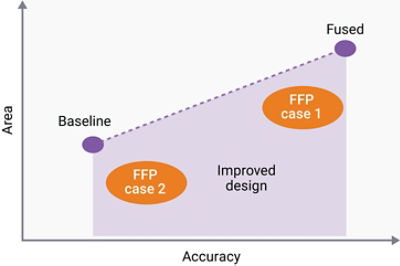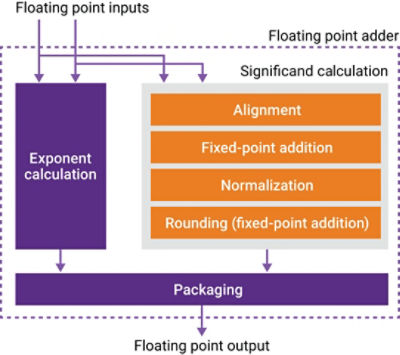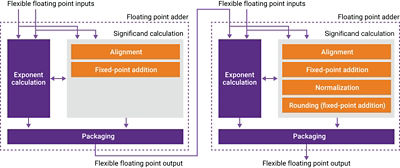# Hardware Mathematics for Artificial Intelligence

By: John A. Swanson, Sr. Product Marketing Manager, Synopsys

Artificial intelligence (AI) has the potential to fundamentally change the way we interact with our devices and live our lives. Petabytes of data efficiently travels between edge devices and data centers for processing and computing of AI tasks. The ability to process real world data and create mathematical representations of this data is a key component, and in some cases, a product differentiator. Accurate and optimized hardware implementations of functions offload many operations that the processing unit would have to execute. As the mathematical algorithms used in AI-based systems stabilize, the demand to implement them in hardware increases which is advantageous for many AI applications freeing compute resources with hardware implementations.

For AI, one of the important concepts to understand is a neural network. A neural network is defined as a computer system modeled on the human brain and nervous system. In hardware, this is a function that will “learn” an output for a given input. Training is “learning”. Showing the network many images of what you are training the network to recognize is implemented using math.

Running a trained neural network to analyze and classify data, calculate missing data, or predict future data is called inference. Inference is implemented by taking the input data, multiplying it with a weighted matrix, and applying an activation function used to determine whether the information that the neuron is receiving is relevant for the given information or if it should be ignored. There are different activation functions that can be used, from simple binary step functions to more complex activation functions like the widely used Sigmoid function, which is described as S(x) = 1/(1+e-x). The Sigmoid function provides an “S” shaped curve where S(x) = 0 as x approaches -∞ and 1 as x approaches +∞, and when x = 0 the output is 0.5, as shown in Figure 1. Values that are greater than 0.5 can be labeled as a ‘yes’ while values that are less than 0.5 can be labeled as a ‘no’, or there is a percentage or probability of “yes/no” using the value of the curve at a given point. One of the limitations of the Sigmoid function is when values are greater than 3 or less than -3, the curve gets quite flat and very high precision is required to continue learning.Figure 1: The Sigmoid function is a widely used activation function for artificial neurons

There is debate over the need for floating point (FP) precision and the complexities it brings into the design. Today’s neural network predictions often don't require the precision of floating point calculations with 32-bit or even 16-bit numbers. In many applications, designers can use 8-bit integers to calculate a neural network prediction and still maintain the appropriate level of accuracy. These present different predicaments when it comes to design requirements for AI applications. For example, is the neural network recognizing all the different shades of blue or just the color blue.

## Controlling Design Precision with IP

The DesignWare® Foundation Cores library of mathematical IP cores provides designers a very flexible set of operations to implement mathematical constructs in AI applications. Optimized for efficient hardware implementation, the DesignWare Foundation Cores allows designers to make tradeoffs in power, performance, and area by controlling their design precision while meeting their unique design requirements. To illustrate this, consider a baseline or simple multiplier compared to a fused or pre-built multiplier capable of handling multiple precisions, as shown in Figure 2.Figure 2: Baseline vs. fused multipliers to handle multiple precisions

For both floating point and integer operations, designers can control internal or inter-stage rounding between the operations. In floating point operations, internal rounding control as well as the flexible floating point (FFP) format enables designers to make more efficient hardware implementations through the technique of sharing common operations. Using the FFP format, designers can implement their own specialized FP components and with bit-accurate C++ models, they can explore the area and accuracy of the components to ensure their design-specific requirements.

Floating point algorithms are combinations of atomic operations. The IP that supports floating point designs is based on sub-functions as part of the floating point algorithms. To identify sub-functions, let’s look at a simple block diagram of a floating-point adder, shown in Figure 3.Figure 3: IP-based floating point design based on sub-functions

There are two main paths in the floating point adder: exponent calculation and significand calculation. Those paths exchange information to generate the final calculated result. When the significand is scaled, the exponent is adjusted accordingly. The main sub-function includes the significand calculation, which are alignment, fixed-point addition, normalization, and rounding. Normalization is a large percentage of the logic that makes up a floating point adder, and if eliminated, it would give margin to hardware optimization. The lack of normalization, however, may cause some loss of significand bits during operations, something that must be considered. Internal values of the significand are represented in the fixed-point, and they are tightly connected with exponent value in the exponent calculation channel. To implement sub-functions, there needs to be a way to carry exponent and fixed-point significand together as it's done in the standard floating-point system, but with fewer constraints and special cases.

In a sequence of floating point additions, the normalization and rounding steps account for a significant portion of the critical path. When creating compound operations, it is usually recommended to leave normalization and rounding to the final stage of a series of operations. This is because direct access to the internal fixed-point values of a floating-point operation, without normalization or rounding, reduces complexity, area, and critical path and it gives designers control over internal precision. A simple example of a two-stage adder is shown in Figure 4.

By eliminating normalization and rounding, the numerical behavior changes. First, the exponent calculation is somewhat simplified in the first FP adder. Second, the representation of our floating-point output does not comply with the IEEE 754 standard anymore. Third, the second floating-point adder in the chain needs to understand this new representation and it needs to have some information about what happened in the previous calculation to make decisions.Figure 4: Compound functions from simpler sub-blocks

Synopsys’ DesignWare Foundation Cores provide a library of components that are used when creating a project that involves IP. As each component is configured, the RTL and C models are installed into a project directory, which includes all the files associated with the verification and implementation of the components. Once the design is complete, the files integrate into the larger SoC design and designers can continue to refine their RTL for the next generation of the product.

## Summary

The explosion of AI is driving a new wave of designs to enable more direct interactions with devices in the real world. The ability to process real world data and create mathematical representations of this data is a key component and designers are trying many different approaches to apply AI to their products. Accurate and optimized hardware implementation of functions offload many operations that the processing unit would have to execute. Implementing mathematical algorithms in hardware becomes advantageous in AI systems.

The DesignWare Foundation Cores gives designers a powerful tool to quickly meet their designs' unique requirements and achieve precision with the ability to explore design space with different configurations and implementations to make accuracy, power, performance, and area trade-offs. With the bit accurate C++ models, designers can quickly run simulations on the generated models to ensure that their AI design meets project requirements.